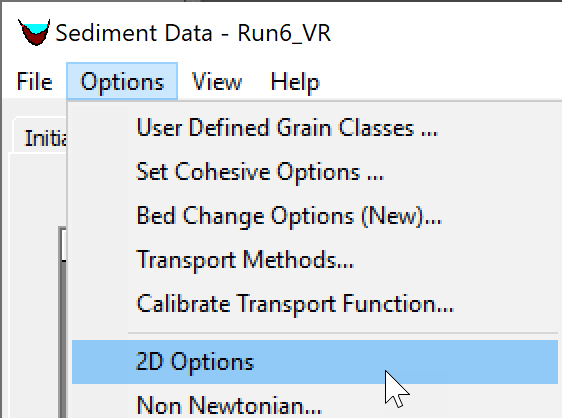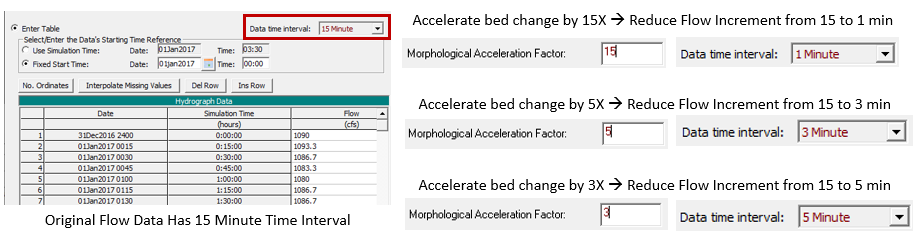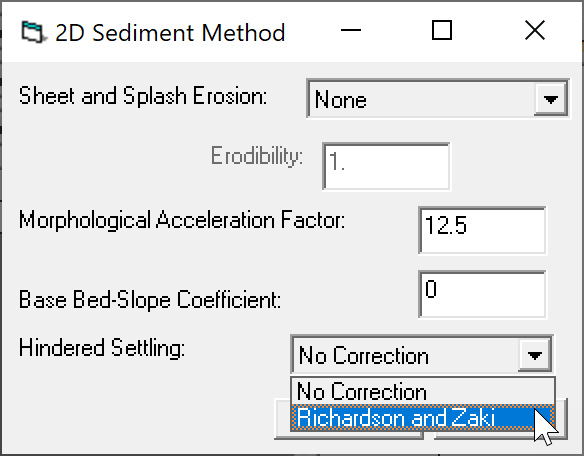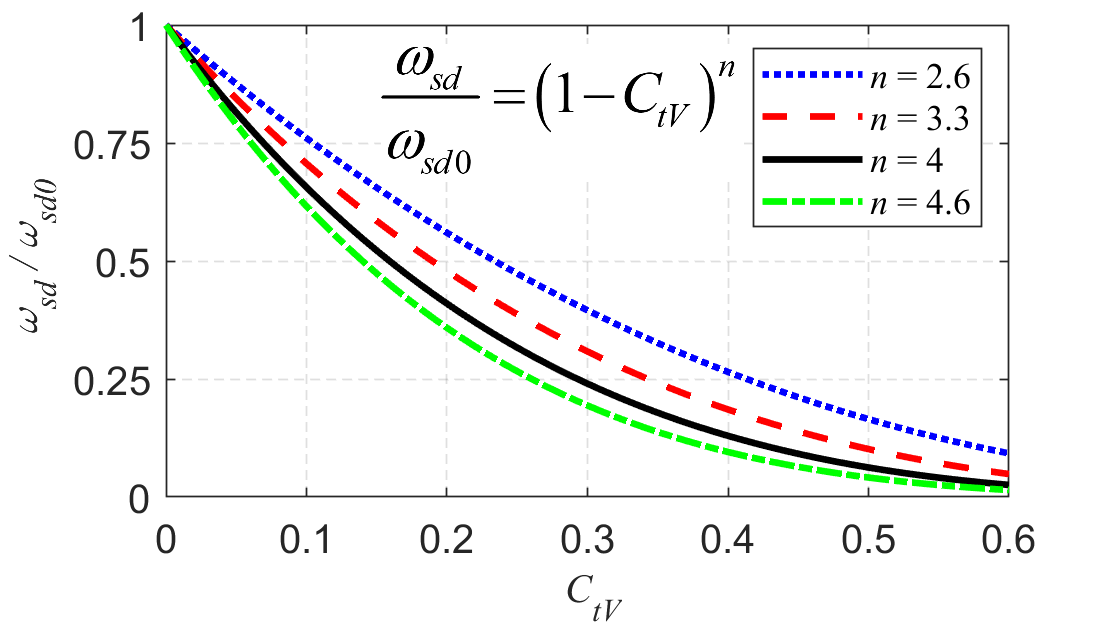There are several sediment transport options which are only available in 2D. The goal is for both 1D and 2D sediment transport to have the same options but for now the 2D only options have been places in a single editor called 2D Options which is available from the Sediment Data editor under the Options menu (see figure below).Figure 1. Opening the 2D Options editor from the Sediment Data editor.

The 2D Sediment Options editor is shown in the figure below. The editor is used to specify the options for sheet and splash erosion, the morphologic acceleration factor, the base bed-slope coefficient, and hindered settling.Figure 2. 2D Options editor.

# Capacity Only Method

In HEC-RAS Version 6.2 the Capacity Only Method was introduced. If the Capacity Only Method is checked on, the model computes only the sediment transport capacity (i.e. equilibrium concentrations) for all grain classes and does not solve the transport, bed sorting, and bed layering equations. The transport rates are computed with the sediment capacity. The Capacity Only Method runs much faster because it does not solve transport, bed sorting, and bed layering equations for each grain class. This makes the method very useful for screening sediment transport functions and estimating initial sediment bed gradations and sediment boundary conditions.

# Sheet and Splash Erosion

The first option available in the editor is the Sheet and Splash Erosion. The 2D sediment transport model has the option to use the Wei et al. (2009). The formulation has an erodibility coefficient which for simplicity is set to a single value in HEC-RAS. The units of the erodibility coefficient are kg∙m-3.644∙s0.644. Wei et al. (2009) reported values for the sheet and splash erosion coefficient between 1124 and 2555 kg∙m-3.644∙s0.644 for 3 grassland rangeland plots in Arizona (all variables in the International System of Units). However, its value can vary by orders of magnitude for different soil types and cover characteristics.

# Morphologic Acceleration Factor

The Morphologic Acceleration Factor can turn off the bed change or - as the name implies - accelerate the bed change. The factor is directly multiplied by the mass bed exchange rates at every time step. A value of zero will turn off the bed elevation and bed composition change.

The Morphologic Acceleration Factor can be utilized in several ways. It can turn off bed change (Morph Acceleration = 0). This saves computational time and is useful for idealized situations are when debugging a problem with a sediment model.  The "Concentration Only" computational mode provides the same capability now, however, and is a more direct method for fixed-bed sediment modeling.

The Morphologic Acceleration Factor is usually a scaling factor for bed change change. The factor can be simulate a time period which represents the morphological change of a time period equal to the simulation period times the Morphologic Acceleration Factor. This approach is commonly used in coastal applications with tidal boundary conditions. As an example, a 5-year simulation can be run with Morphologic Acceleration Factor of 20 to simulate 100 years of change, which greatly reduces the computational time. However, it should be noted that this approach changes the order of events (i.e. storms and tidal forcing with respect to morphological features) which can have a negative impact on the accuracy of the approach.

Lastly, the Morphologic Acceleration Factor can speed-up run time. This approach is more appropriate in river applications or when simulating single events or sequences of events. As an example, a 20-day simulation can be reduced to 2 days by setting the Morphologic Acceleration Factor to 10.  In this approach you also must speed up the boundary conditions by the same factor. However, do not speed-up the time so much that the hydrodynamics change substantially and thereby the morphological change.Changing the Boundary Condition Time Step with the Morphological Acceleration Factor

It is useful to choose a Morphological Acceleration Factor that is easily divisible by the time step.  For example, if a boundary condition data increment is 15 minutes, selecting Morphological Acceleration Factors of 3, 5, or, 15 makes it easier to adjust these data to accommodate the temporal dilation.  See these three examples below:The Morphologic Acceleration Factor should be used with caution as it can lead to misleading results or instabilities. The best practice for using the factor is to test the validity of the factor by doing a full or partial length of a simulation with the factor and without it and compare the results. If the results agree reasonably well, then longer or alterative simulations can usually be done with the Morphologic Acceleration Factor thus saving time. It is generally not recommended to use a factor larger than 30 to 50, and values between but not equal to 0 and 1. The Morphologic Acceleration Factor can be verified by reducing its value (e.g. by a factor of 2) while also increasing the simulation time by the same factor and verifying the simulation results do not significantly change.

Since, the Morphologic Acceleration Factor is applied to the bed exchange rates, the approach does not change total-load transport rates or concentrations. In addition, the approach is not locally mass conservative but approximately globally conservative.

Finally, it is important to emphasis that the Morphologic Acceleration Factor should NOT be used as a calibration parameter. It should be used carefully and validated to make sure the results are not sensitive to the Morphologic Acceleration Factor. When used carefully the Morphologic Acceleration Factor can be a powerful and useful tool in numerical studies.

# Base Bed-Slope Coefficient

The Base Bed-Slope Coefficient specifies the maximum value of the bed-slope coefficient. The coefficient is then reduced based on the skin and critical shear stresses. As the ratio between the skin and critical shear stress increases bed-load particles are less influenced by the bed slope. The coefficient is used to compute an additional sediment flux in the downslope direction which is a function of the bed slope, the bedload, and the bed-slope coefficient. For further details on the formulation, the user is referred to the HEC-RAS 2D Sediment Technical Reference manual (HEC 2020). The Base Bed-Slope Coefficient typically has a value between 0.1 and 1. Increasing the coefficient has the effect of smoothing the bathymetry. Measured bed change can be used to calibrate the base bed-slope coefficient. However, its effect is significantly less than the transport formula, transport scaling factors and mobility scaling factor. The Base Bed-Slope Coefficient will tend to improve model stability by smoothing out small scale instabilities. However, if the value is too large the numerical scheme can become unstable. In these situations the user can either reduce the computational time step or reduce the value of the Base Bed-Slope Coefficient.Modeling Note: Base Bed-Slope Coefficient Range

The Base Bed-Slope Coefficient generally varies from 0.1 to 0.5 and higher values tend to smooth results.

# Hindered Settling

Hindered settling is the condition in which the settling velocity of particles or flocs is reduced due to a high concentration of particles. Hindered settling is primarily produced by particle collisions and the upward water flow equal to the downward sediment volume flux. Hindered settling occurs to both cohesive and noncohesive particles. However, the hindered settling correction described here only applies to noncohesive particles. The hindered settling of cohesive particles is accounted for in the floc settling method. Currently, the 2D sediment has the option to use the Richardson and Zaki (1954) formula for hindered settling (see figure below). For simplicity the exponent in the Richardson and Zaki (1954) is set to 4.0 and cannot be modified in the user interface.Figure 3. Setting the hindered settling method to the Richardson and Zaki (1954) method.

The figure below shows the ratio of the hindered settling velocity $//$ and free settling velocity $//$ as a function of the total sediment concentration by volume following Richardson and Zaki (1954).Figure 4. Richardson and Zaki (1954) hindered settling velocity formula.

# Avalanching

HEC-RAS Version 6.2 and newer support the option to simulate sediment sliding or avalanching. This option is limits the bed slope to the angle of repose. A single angle of repose is utilized for both wet and dry cells. The angle of repose can vary from about 30º to nearly vertical for cohesive beds. Avalanching is computed with an iterative relaxation approach which has two parameters: (1) the maximum number of iterations, and (2) a relaxation factor. The recommended range for the maximum number of iterations is between 5 to 20. The range for the relaxation parameter is typically between 0.1 and 0.3. The angle of repose, maximum number of iterations, and relaxation factor are specified in the Avalanching section of the 2D Options menu.